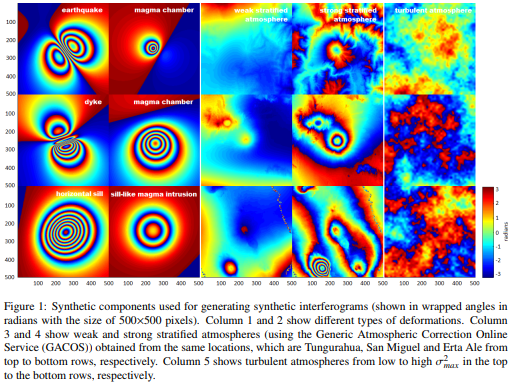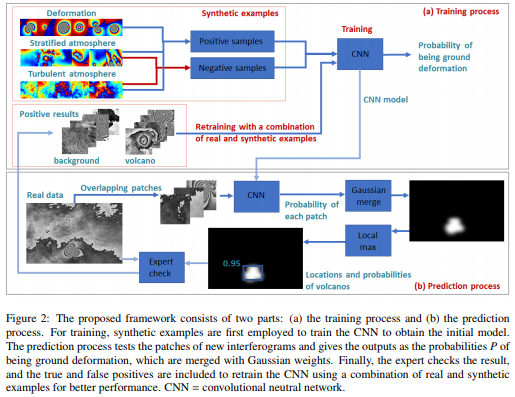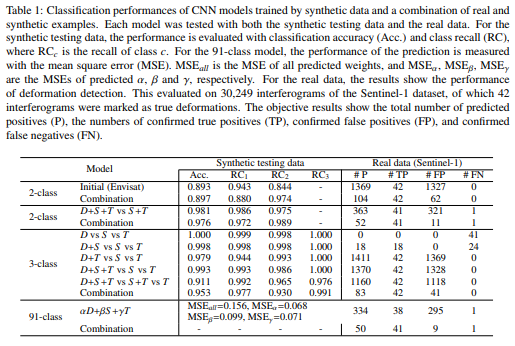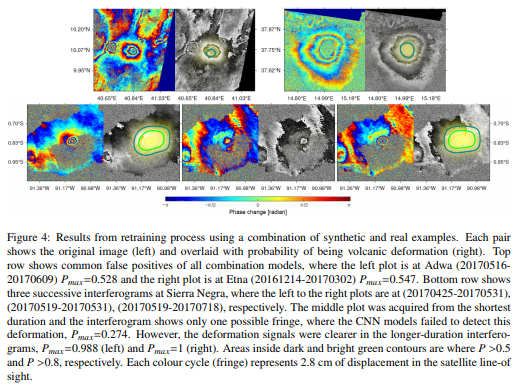They used Interferometric Synthetic Aperture Radar (InSAR), to detect surface deformation with a strong statistical link to eruption. As only a small proportion of volcanoes are deforming the atmosphere (usually during an eruption), the use of deep learning for detecting volcanic unrest is more challenging than many other applications. In this paper, they address this problem using synthetic interferograms to train the AlexNet CNN. The synthetic interferograms are composed of 3 parts:

• Deformation patterns based on a Monte Carlo selection of parameters for forwarding analytic models.
• Stratified atmospheric effects derived from weather models.
• Turbulent atmospheric effects based on statistical simulations of correlated noise.

They demonstrate that training with synthetic examples can improve the ability of CNNs to detect volcano deformation in satellite images, and propose an efficient workflow for the development of automated systems.

## Imbalanced training data and solutions

Features of the minority class are treated as noise and often ignored. In InSAR datasets, the number of interferograms showing deformation in an image is approximately 0.15% of all acquired interferogram, and the ratio of positive and negative areas is only 1:15,000. The global dataset covered over 900 volcanoes in 2016-2017, but only 4 volcanoes are in activity during that time (Cerro Azul, Sierra Negra, Etna, and Erta Ale). This means that all existing methods would lead to overfitting using InSAR. Therefore, they propose generating synthetic data to improve classification performance.

## Generation of Synthetic Training Data

They use existing models to create synthetic examples of

• deformation
• stratified atmospheric artifacts
• turbulent atmospheric artifacts

Monte Carlo is used to approaching the selection of parameter values, thereby including scenarios that are considered feasible but have not observed. The resulting synthetic training datasets should provide a better generalization than the real dataset. InSAR produces maps of phase change between two time-separated radar images. The phase shift is a combination of

• satellite viewing geometry
• instrument thermal noise
• atmospheric delay
• systematic changes to dielectric properties
• scattering properties of a pixel
• surface deformation

They generate the 10 000 synthetic images for each of the 3 components, deformation D, stratified atmosphere S, and turbulent atmosphere T.## Method

Initially, they train the network using just the synthetic images. They are labeled as 1 or positive, where deformation included, and 0 or negative in other combinations. Then, the initial model employed in the prediction process, where the new interferogram divided into overlapping patches and those containing phase jumps are tested with the trained CNN model. An expert then checks the results, the model is retrained, and the classification is repeated.• 2-class model: The model is trained with 2 classes: deformation and non deformation. They generated the training data by combining signals D+S+T for the deformation class and only S+T for the non deformation class. For eachcombined signal, the components D, S and T are randomly selected.
• 3-class model: Initially they trained the CNN with completely separate D, S and T signals, but this is a poor representation of real datasets, so we also trained the classifier with several more realistic combinations (e.g. D+S, D+T, D+S+T).
• 91-class model: They generated weighted interferograms (I) by combining three components as $$I=(\alpha D+\beta S+\gamma T)/(\alpha+\beta+\gamma)$$, where $$(\alpha,\beta,\gamma)\in[0,0.25,0.5,0.75,1]$$. Varying three weights with five values creates 91 unique combinations, resultingin 91 classes: class 1 is $$[\alpha_1=0,\beta_1=0,\gamma_1=1]$$; class 2 is $$[\alpha_2=0,\beta_2=0.25,\gamma_2=0.75]$$; …; class 91 is $$[\alpha_{91}=1,\beta_{91}=1,\gamma_{91}=1]$$. They applied a weight estimation approach using a multinomial classification.

## ResultsSentinel-1 takes data from the same place between 6 to 12 days, and an eruption have a time activity around 7 weeks